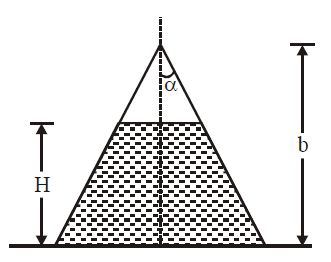# Upward Lifting force on a Cone!

A conical cup of height $b$, semi-vertical angle $\alpha$ rests open end down on a flat surface as shown. The cup is filled to height $H$ with liquid of density $\rho$ and a small hole is punched at the apex of the cone. The upward lifting force on the cup is $F= \pi \rho g [bH^2 - \frac{H^3}{n}] \tan^2 \alpha$ , then '$n$' is×